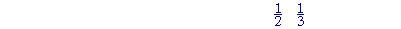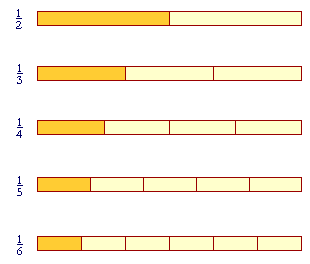S k i l l
i n
A R I T H M E T I C

Lesson 20  Section 2

# Comparing fractions

Back to Section 1

 10. What is the relative size of fractions that have equal numerators?The larger the denominator, the smaller the fraction.Those fractions are getting smaller.  As the denominator -- the number of equal parts -- gets larger, then the size of each part gets

 smaller. 16 is smaller than 15 .

Also, since one-sixth is smaller than one-fifth, then two will be smaller than two:

 2 6 is smaller than 25 .

Three will be smaller than three:

 3 6 is smaller than 35 .

And so on.

When frations have equal numerators, then the larger the denominator,
the smaller the fraction.

 23 , 24 , 25 , 26 .

Those fractions are getting smaller.

In terms of ratios, the ratio of 1 to 2, for example, is greater than the ratio of 1 to 3:When we compare 1 with 2, it appears greater than when we compare it with 3.

 11. What is the relative size of fractions that have equal denominators?The larger the numerator, the larger the fraction.

In this sequence,

 26 , 36 , 46 , 56 ,

the fractions are getting larger.  Each one is one more of the 6 equal parts into which number 1 has been divided.

As for ratios, we say that the ratio of 2 to 5 is smaller than the ratio of 3 to 5:2, when compared with 5, appears smaller than 3 when compared with 5.

Example 1.   Arrange these from smallest to largest:

 57 49 47
 Solution.  We must compare them in pairs. 49 and 47 have the same

numerator; therefore

 49 is smaller than 47 .
 57 and 47 have the same denominator; therefore
 57 is larger than 47 .

The sequence is

 49 47 57 .

 Example 2.   Which is smaller, 1 10 or 29 ?
 Answer.  Since 1 10 is smaller than 19 , then it is surely smaller than 29 .

In Lesson 23 we will see how to compare fractions with different numerators and denominators.

Please "turn" the page and do some Problems.

or

Continue on to the Section 3.

Section 1 on Fractions